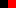T H E   S T Y L E   T O   C A R R YP R O D U K T EC H E C K L I S T ER E F E R E N Z E NA N F R A G EK O N T A K TH O M E